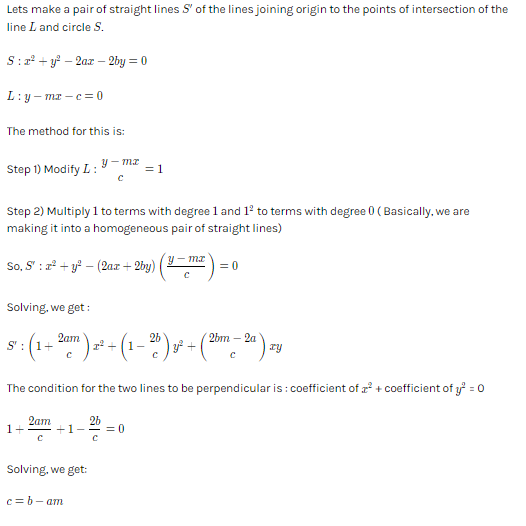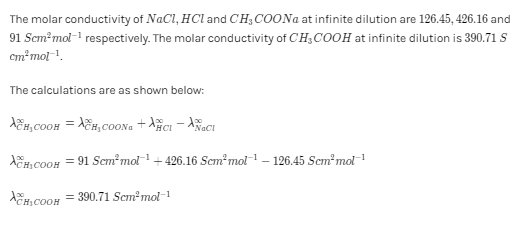# Find the equation to the straight lines joining the origin to the points in which the straight line y= mx+c cuts the circle, x2+y2= 2ax+2by. Hence , find the condition that these points may subtend a right angle at origin. Also find the condition that the straight line may touch the circle.Saurabh Koranglekar